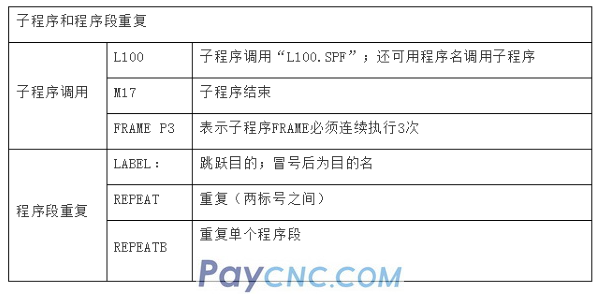1. The H code of FANUC—0M macro program Macro standard format: G65 Hm P#i Q#j R#k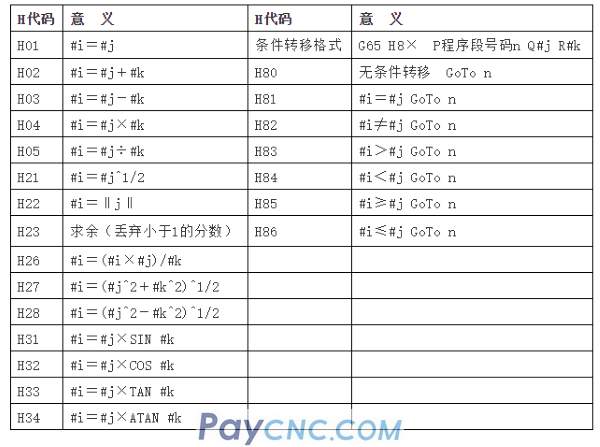2. The format of FANUC—18M macro program 1. Types of variables: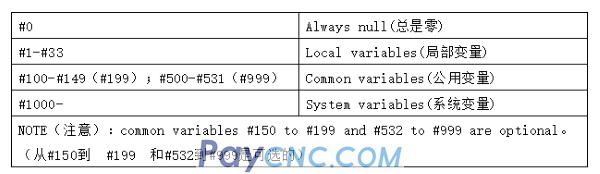2. Non-modal call macro program: The standard format of the program: G65 P (macro program number) L (number of repetitions) Note: The standard format of G65 must be rewritten every time it is called, and it will be cancelled automatically after use. 3. Modal call macro program: The standard format of the program: G66 P (macro program number) L (number of repetitions) Note: Input parameter value directly without specifying G66. 4. About the parameter assignment in G65 and G66 ⑴. Correspondence between the address of parameter assignment Ⅰ and the variable number: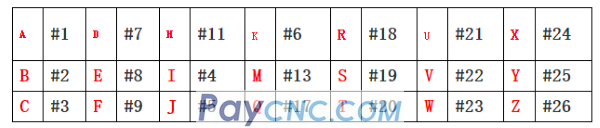⑵. Correspondence between the address of parameter assignment Ⅱ and the variable number: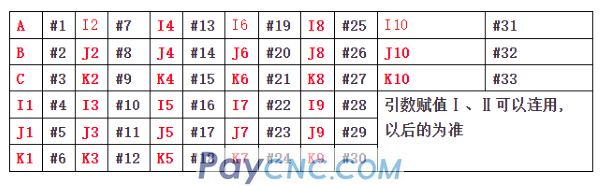5. Regarding the arithmetic and logical operations in G65 and G66: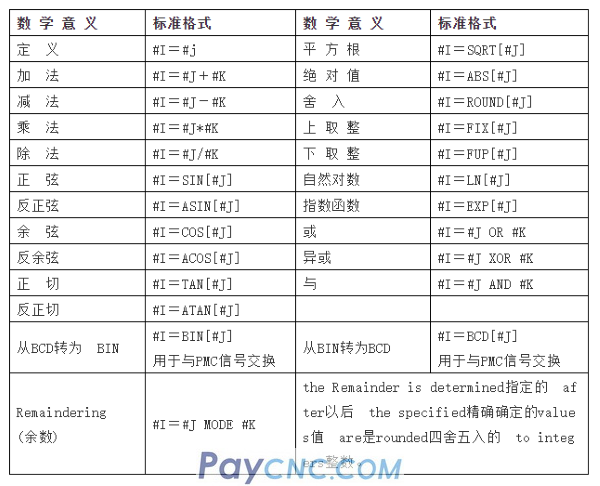6. The modal call of G67 macro program cancels the macro program. 7. The macro program can be called in the format of G××　 : Description: ①The program number uses O9010~O9019; ②Set the parameter corresponding to the program number to the value of G code; ③The calling form is: G (parameter setting value) . The main body number of the macro program and the corresponding parameter number: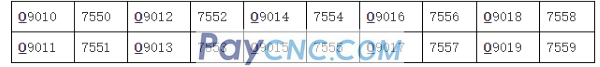8. Conditional transfer instructions: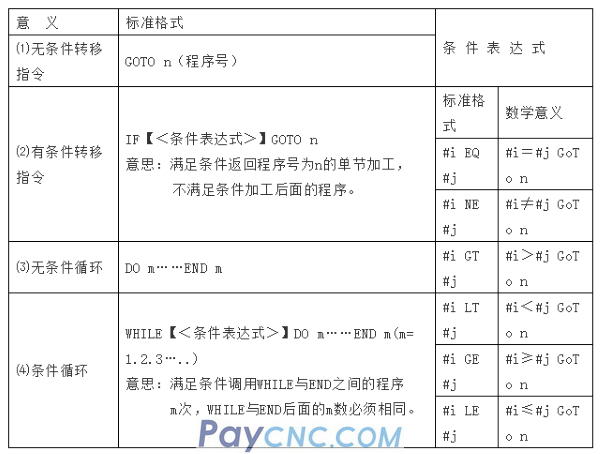3. The format of the SIEMENS macro program 1. Calculate the parameter R: R0—R99 —— can be used freely; R100R249 ——Machining cycle transfer parameters; R250—R299—Internal calculation parameters used for processing cycle transmission. Calculation: Follow the usual rules of mathematical operations, priority in parentheses, priority in multiplication and division, and addition and subtraction. For example: R15=SQRT(R1*R1+R2*R2); meaning: R15=(R1^2+R2^2)^1/2; N10 Z=R3 means Z assignment; the rest is the same as 18M. 2. Conditional jump: ⑴. Standard format: IF condition GoToF Label jump forward IF condition GoToB Label jump backward   Among them: label (Label): the two characters starting with 2-8 letters or numbers must be letters or underscores. The jump target identifier must be followed by a colon. ⑵. Comparison operation: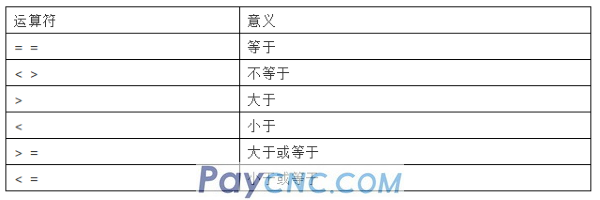3. Arithmetic:Fourth, the format of the SIEMENS programming language